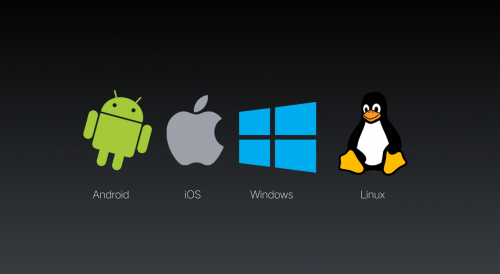##### 使用信号量实现
###### 读者优先

``````void writer
{
while(true)
{
P(wt);
write();
V(wt);
}
}
{
while(true)
{
P(mutex)           //对rcount进行互斥访问
rcount++;
if(rcount==1)
P(wt)          //如果是第一个读者，与写者互斥抢占数据库
V(mutex)

P(mutex)          //对rcount进行互斥访问
rcout--;
if(rcount==0)
V(wt)         //如果是最后一个读者，释放数据库所有权
V(mutex)
}
}``````

###### 写者优先

``````void reader()
{
while(true)
{
P(rdmutex)           //对rcount进行互斥访问
if(rcount ==0)       //如果是第一个读操作，与写者互斥抢占数据库
P(wt)
rcoutn++;
V(rdmutex)
V(x)
P(rdmutex)           //对rcount进行互斥访问
rcount--;
if(rcount==0)        //如果是最后一个读者，释放数据库所有权
V(wt)
V(rdmutex)
}
}
void writer()
{
while(true)
{
P(wtmutex)
if(0 == wcount)
wcount++;
V(wtmutex)
P(wt)
write()  //写
V(wt)
P(wtmutex)
wcount--;
if(wcount==0)